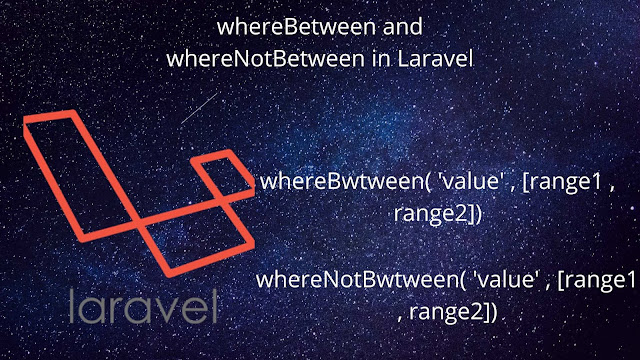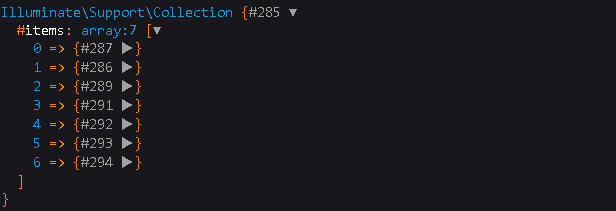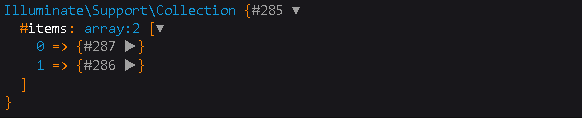# Discovering Laravel Eloquent: Unleash whereBetween Magic!

0

## whereBetween and whereNotBetween Query in LaravelIn this article we will see how can we use whereBetween and whereNotBetween method in Laravel and how it is different from normal SQL query .

whereBetween method basically takes two parameter one is the column_name and another is an array with two values that is start_range and end_range and loop through your database table and collect all the rows in the format of an array that falls inside the range .

Similarly whereNotBetween method also takes two parameter same as whereBetween method that are column_name and an array having two values with start_range and end_range . It collects all the rows that does not fall under the range .

You can also read my article on how to use multiple where condition in Laravel .

Let's see some practical Examples for better understanding .

### Laravel Eloquent whereBetween :

#### SQL Query :

`SELECT * FROM products`
```
WHERE price BETWEEN 1 AND 100;
```

#### Laravel Syntax 1:

```\$data=Model_Name::select('*')

->whereBetween('column_name',[start_range,end_range])

->get();

dd(\$data);
```

#### Laravel Example 1:

```\$data=Product::select('name')

->whereBetween('price',[1,100])

->get();

dd(\$data);
```
```
```

#### Laravel Syntax 2:

```\$data=DB::table('table_name')

->whereBetween('column_name',[start_range,end_range])

->get();

dd(\$data);
```

#### Laravel Example 2:

```\$data=DB::table('products')

->whereBetween('price',[1,100])

->get();

dd(\$data);
```

### Output :### Laravel Eloquent whereNotBetween :

#### SQL Query :

`SELECT * FROM products`
```WHERE price NOT BETWEEN 50 AND 100;
```

#### Laravel Syntax 1:

```\$data=Model_Name::select('*')

->whereNotBetween('column_name',[start_range,end_range])

->get();

dd(\$data);
```

#### Laravel Example 1:

```\$data=Product::select('name')

->whereNotBetween('price',[50,100])

->get();

dd(\$data);
```

#### Laravel Syntax 2:

```\$data=DB::table('table_name')

->whereNotBetween('column_name',[start_range,end_range])

->get();

dd(\$data);
```

#### Laravel Example 2:

```\$data=DB::table('products')

->whereNotBetween('price',[50,100])

->get();

dd(\$data);```

### Output :So these are the ways that you can use whereBetween and whereNotBetween method .

Hope it helped you .

For any query do not hesitate to comment 💬

Get average in Laravel Eloquent

Search functionality in Laravel

Laravel 7/6 Email Verification

Why Laravel is so Popular PHP Framework

Laravel 7 PDF Generation ( DomPDF )